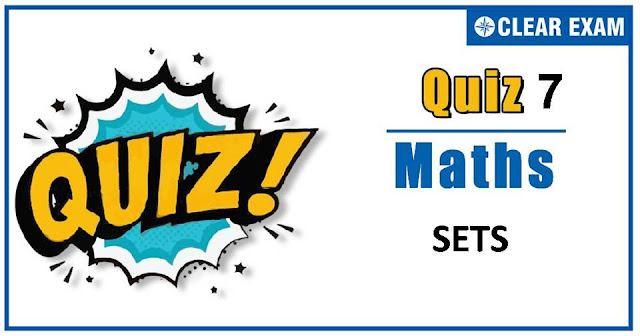## [LATEST]\$type=sticky\$show=home\$rm=0\$va=0\$count=4\$va=0

As per analysis for previous years, it has been observed that students preparing for JEE MAINS find Mathematics out of all the sections to be complex to handle and the majority of them are not able to comprehend the reason behind it. This problem arises especially because these aspirants appearing for the examination are more inclined to have a keen interest in Mathematics due to their ENGINEERING background.

Furthermore, sections such as Mathematics are dominantly based on theories, laws, numerical in comparison to a section of Engineering which is more of fact-based, Physics, and includes substantial explanations. By using the table given below, you easily and directly access to the topics and respective links of MCQs. Moreover, to make learning smooth and efficient, all the questions come with their supportive solutions to make utilization of time even more productive. Students will be covered for all their studies as the topics are available from basics to even the most advanced.

Q1. Two points P and Q in a plane are related if OP=OQ, where O is a fixed point. This relation is
•  Partial order relation
•  Equivalence relation
•  Reflexive but not symmetric
•  Reflexive but not transitive
Solution
No solution available

Q2.If R be a relation < from A={1,2,3,4} to B={1,3,5} i.e.(a,b)∈R⇔a < b, then R o R-1 is
•  {(1,3),(1,5),(2,3),(2,5),(3,5),(4,5)}
•  {(3,1),(5,1),(3,2),(5,2),(5,3),(5,4)}
•  {(3,3),(3,5),(5,3),(5,5)}
•  {(3,3),(3,4),(4,5)}
Solution
We have, R={(1,3),(1,5),(2,3),(2,5),(3,5),(4,5)} ⇒R-1={(3,1),(5,1),(3,2),(5,2),(5,3),(5,4)} Hence, R o R-1={(3,3),(3,5),(5,3),(5,5)}

Q3.  Let A and B be two sets, then (A∪B)'∪(A'∩B)is equal to
•  A'
•  A
•  B'
•  None of these

Q4. If A={x,y,z}, then the relation
R={(x,x),(y,y),(z,z),(z,x),(z,y)} is
•  Symmetric
•  Antisymmetric
•  Transitive
•  Both (a) and (b)
Solution
Clearly, R is an equivalence relation on A

Q5.If A={1,2,3,4,5,6}, then how many subsets of A contain the elements 2,3 and 5?
•  4
•  8
•  16
•  32
Solution
The number of subsets of A containing 2, 3 and 5 is same as the number of subsets of set {1,4,6} which is equal to 23=8

Q6. Let A be the set of all students in a school. A relation R is defined on A as follows:
"aRb iff a and b have the same teacher”
•  Reflexive
•  Symmetric
•  Symmetric
•  Equivalence
Solution

Q7.Let R be a relation defined on S, the set of squares on a chess board such that xRy iff x and y
share a common side. Then, which of the following is false for R?
• Reflexive
•  Symmetric
•  Transitive
•  All of the above
Solution
Clearly, R is reflexive and symmetric but it is not transitive

Q9.Which one of the following relations on R is an equivalence relation?
•  a R1 b⇔|a|=|b|
•  a R2 b⇔a≥b
•  a R3 b⇔a divides b
•  a R4 b⇔a< b
Solution
No solution available

Q10. If n(A×B)=45, then n(A) cannot be
•  15
•  17
•  5
•  9
Solution
We have, n(A×B)=45 ⇒n(A)×n(B)=45 ⇒n(A)and n(B) are factors of 45 such that their product is 45 Hence, n(A) cannot be 17#### Written by: AUTHORNAME

AUTHORDESCRIPTION## Want to know more

Please fill in the details below:

## Latest NEET Articles\$type=three\$c=3\$author=hide\$comment=hide\$rm=hide\$date=hide\$snippet=hide

Name

ltr
item
BEST NEET COACHING CENTER | BEST IIT JEE COACHING INSTITUTE | BEST NEET & IIT JEE COACHING: SETS-QUIZ-7
SETS-QUIZ-7
https://1.bp.blogspot.com/-l25z6fdxNM4/X5ZRK3UE5eI/AAAAAAAAFjg/TfK0-WSZPwUb3X7-Ipc88NWYiuKchI7VgCLcBGAsYHQ/w640-h336/quiz.jpg
https://1.bp.blogspot.com/-l25z6fdxNM4/X5ZRK3UE5eI/AAAAAAAAFjg/TfK0-WSZPwUb3X7-Ipc88NWYiuKchI7VgCLcBGAsYHQ/s72-w640-c-h336/quiz.jpg
BEST NEET COACHING CENTER | BEST IIT JEE COACHING INSTITUTE | BEST NEET & IIT JEE COACHING
https://www.cleariitmedical.com/2020/10/SETS-QUIZ-7.html
https://www.cleariitmedical.com/
https://www.cleariitmedical.com/
https://www.cleariitmedical.com/2020/10/SETS-QUIZ-7.html
true
7783647550433378923
UTF-8

STAY CONNECTED